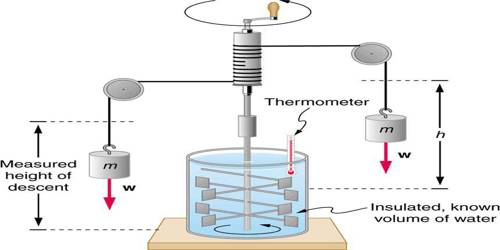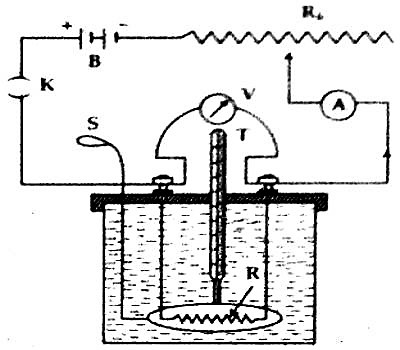Physics

# Experiment: determine the Mechanical Equivalent of HeatExperiment: to determine the mechanical equivalent of heat, J

There are two electrical methods for the determination of mechanical equivalent of heat, viz – (i) Joule’s method and (2) Calender and Berns’s method. Here Joule’s method is discussed below.

Principle or Theory: We know, the amount of work done due to the flow of current ‘i’ for ‘t’ is through a conductor having potential difference of V volt,

W =Vit J … … (1)

If this heat, being converted to heat energy, produces H calorie heat, then we get,

W = J H … … (2)

From equations (1) and (2) we get,

JH = Vit

or, J = (Vit / H) J cal-1

Working principle: Let us take a dean and dry Joule’s calorimeter and weight it in an empty condition. Now let us take some liquid (water or tarpin oil) in the calorimeter and again take the weight. From the difference of the above two weights let us find the mass of the liquid. Then the initial temperature of the calorimeter and the liquid is measured by a thermometer T.

Now, by inserting a coil of resistance of R ohm inside the liquid a battery B, variable resistor Rh, ammeter A and plug key K are connected by binding screws in series between the terminals of the resistor. To measure the potential difference between the two terminals of the coil a voltmeter V is connected across the coil in parallel [Figure].Now let us close the key and a fixed current is passed in the circuit for certain time (say 15 minutes). During the flow of current readings of the voltmeter and ammeter are taken Heat produced due to the flow of current will be absorbed by the calorimeter and the liquid and their temperatures will rise. For uniform temperature in the liquid the stirrer is moved vigorously and then the maximum temperature of the calorimeter and liquid is measured by a thermometer.

Table: